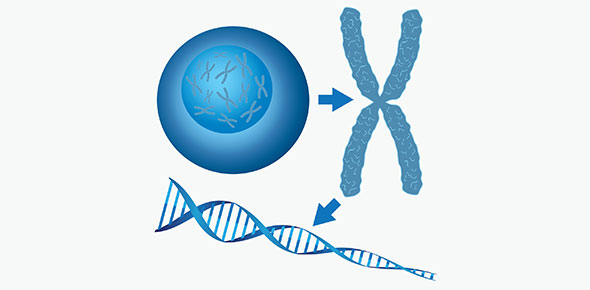7e1 Transformation And Graphs Quiz

10 Questions | Total Attempts: 41Settings.

• 1.
When rotating a shape, the shape always stays the same size.
• A.

True

• B.

False

• 2.
The gradient of the line y = 5x + 1 is ______
• 3.
Rotating a shape by a three quarter turn is how many degrees?
• A.

90

• B.

260

• C.

270

• D.

180

• 4.
What do you need to know to create a rotation?
• A.

Size of Rotation

• B.

Direction of Rotation

• C.

Centre of Rotation

• D.

All of the above

• 5.
When translating a shape, the shape gets bigger
• A.

True

• B.

False

• 6.
Enlarging a shape by a Fractional scale factor, makes the shape ________
• 7.
Which of these co-ordinates is not on the line x =3?
• A.

(2,3)

• B.

(3,2)

• C.

(3,4)

• D.

(3,1)

• 8.
Which of these co-ordinates is not on the line y = x?
• A.

(4,4)

• B.

(3,3)

• C.

(3,2)

• D.

(4,4)

• 9.
Vector notation is used for reflection
• A.

True

• B.

False

Related TopicsBack to top